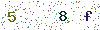﻿ Why should choose a constant current power supply for the LED driver? - LISUNLISUN

# Why should choose a constant current power supply for the LED driver?

The LED is a light-emitting diode, and its characteristic curve is basically the same as that of a normal diode. When the applied voltage reaches the diode conduction voltage, the slight change of the applied voltage can cause a great change in the diode current. The constant current is used to ensure that the current in the LED is constant. No matter how the voltage changes, the current flowing through the LED does not change, which can ensure the life and light decay of the LED.

If a constant voltage source with an output of 12V is used, an electric 16 ohm resistor is connected in series to supply three strings of 0.5W 5630 lamp beads. The lamp voltage is calculated according to 3.3V, the current flowing through the lamp bead Io=(12-3*3.3)/16=0.131A, and the power of a single lamp bead is P=0.131*3.3=0.4323W. Because the temperature of the lamp bead is high The voltage of a single lamp drops to 3V. At this time, the current Io=(12-3*3)/16=0.187A, the current increases by 56 mA., and the power becomes P=0.187*3=0.561 W, which exceeds the lamp power consumption. In the long run, it has a great influence on the life and light decay of LED lamp beads.

Therefore, the key to affecting the life of the LED is the temperature. When the LED temperature rises, the junction resistance decreases, that is, the conduction voltage decreases. At the same voltage, an increase in temperature will cause the LED current to increase, and the current will increase the temperature further, so that the vicious cycle will burn the LED. Moreover, the light decay of LEDs is also related to temperature. When the temperature rises, the light decay is intensified, and the phenomenon of bright and bright is appearing.

There are two reasons for the temperature rise: First, the quality problem, the thermal conductivity of the LED package is poor, the temperature in the LED die is not transmitted to the surface (internal heat and cool), even if the heat sink is added, the internals cannot be completely The heat is dissipated, and then some low-end products do not add a heat sink; the second is the temperature rise caused by the power supply. When the LED is turned on, due to its nonlinearity, the weak change of the power supply will cause a sharp change in the LED current, resulting in temperature.